Alakh Sethi — March 6, 2020

### What is One-Hot Encoding? When should you use One-Hot Encoding over Label Encoding?

These are typical data science interview questions every aspiring data scientist needs to know the answer to. After all, you’ll often find yourself having to make a choice between the two in a data science project!

Machines understand numbers, not text. We need to convert each text category to numbers in order for the machine to process them using mathematical equations. Ever wondered how we can do that? What are the different ways?This is where Label Encoding and One-Hot Encoding come into the picture. We’ll discuss both in this article and understand the difference between them.

Note: Starting your machine learning journey? I recommend taking our comprehensive and popular Applied Machine Learning course!

1. What is Categorical Encoding?
2. Different Approaches to Categorical Encoding
1. Label Encoding
2. One-Hot Encoding
3.  When to use Label Encoding vs. One-Hot Encoding?

## What is Categorical Encoding?

Typically, any structured dataset includes multiple columns – a combination of numerical as well as categorical variables. A machine can only understand the numbers. It cannot understand the text. That’s essentially the case with Machine Learning algorithms too.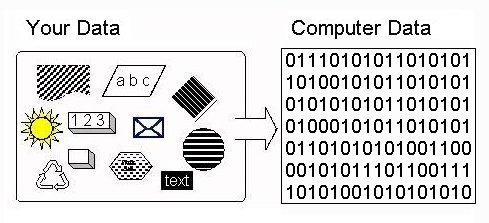That’s primarily the reason we need to convert categorical columns to numerical columns so that a machine learning algorithm understands it. This process is called categorical encoding.

Categorical encoding is a process of converting categories to numbers.

In the next section, I will touch upon different ways of handling categorical variables.

## Different Approaches to Categorical Encoding

So, how should we handle categorical variables? As it turns out, there are multiple ways of handling Categorical variables. In this article, I will discuss the two most widely used techniques:

• Label Encoding
• One-Hot Encoding

Now, let us see them in detail.

### Label Encoding

Label Encoding is a popular encoding technique for handling categorical variables. In this technique, each label is assigned a unique integer based on alphabetical ordering.

Let’s see how to implement label encoding in Python using the scikit-learn library and also understand the challenges with label encoding.

Let’s first import the required libraries and dataset:

Output: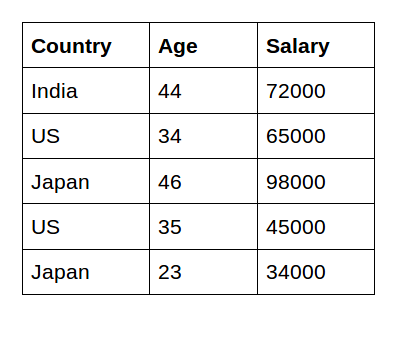Understanding the datatypes of features:

Output: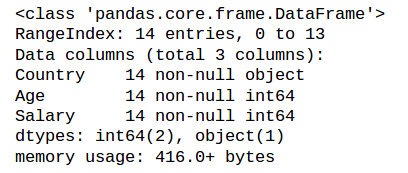As you can see here, the first column, Country, is the categorical feature as it is represented by the object data type and the rest of them are numerical features as they are represented by int64.

Now, let us implement label encoding in Python:

Output: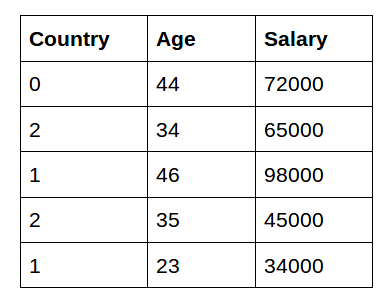As you can see here, label encoding uses alphabetical ordering. Hence, India has been encoded with 0, the US with 2, and Japan with 1.

#### Challenges with Label Encoding

In the above scenario, the Country names do not have an order or rank. But, when label encoding is performed, the country names are ranked based on the alphabets. Due to this, there is a very high probability that the model captures the relationship between countries such as India < Japan < the US.

This is something that we do not want! So how can we overcome this obstacle? Here comes the concept of One-Hot Encoding.

### One-Hot Encoding

One-Hot Encoding is another popular technique for treating categorical variables. It simply creates additional features based on the number of unique values in the categorical feature. Every unique value in the category will be added as a feature.

One-Hot Encoding is the process of creating dummy variables.

In this encoding technique, each category is represented as a one-hot vector. Let’s see how to implement one-hot encoding in Python:

Output: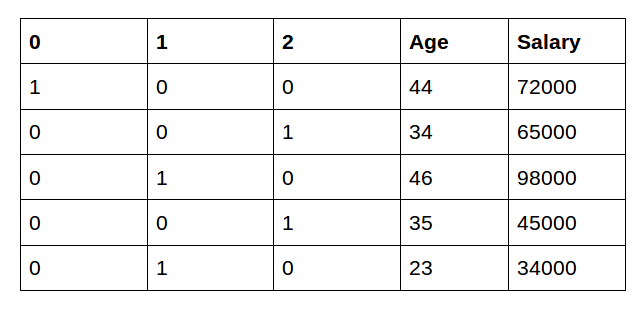As you can see here, 3 new features are added as the country contains 3 unique values – India, Japan, and the US. In this technique, we solved the problem of ranking as each category is represented by a binary vector.

Can you see any drawbacks with this approach? Think about it before reading on.

### Challenges of One-Hot Encoding: Dummy Variable Trap

One-Hot Encoding results in a Dummy Variable Trap as the outcome of one variable can easily be predicted with the help of the remaining variables.

Dummy Variable Trap is a scenario in which variables are highly correlated to each other.

The Dummy Variable Trap leads to the problem known as multicollinearity. Multicollinearity occurs where there is a dependency between the independent features. Multicollinearity is a serious issue in machine learning models like Linear Regression and Logistic Regression.

So, in order to overcome the problem of multicollinearity, one of the dummy variables has to be dropped. Here, I will practically demonstrate how the problem of multicollinearity is introduced after carrying out the one-hot encoding.

One of the common ways to check for multicollinearity is the Variance Inflation Factor (VIF):

• VIF=1, Very Less Multicollinearity
• VIF<5, Moderate Multicollinearity
• VIF>5, Extreme Multicollinearity (This is what we have to avoid)

Compute the VIF scores:

Output: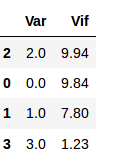From the output, we can see that the dummy variables which are created using one-hot encoding have VIF above 5. We have a multicollinearity problem.

Now, let us drop one of the dummy variables to solve the multicollinearity issue:

Output: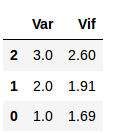Wow! VIF has decreased. We solved the problem of multicollinearity. Now, the dataset is ready for building the model.

I would recommend you to go through Going Deeper into Regression Analysis with Assumptions, Plots & Solutions for understanding the assumptions of linear regression.

We have seen two different techniques – Label and One-Hot Encoding for handling categorical variables. In the next section, I will touch upon when to prefer label encoding vs. One-Hot Encoding.

## When to use a Label Encoding vs. One Hot Encoding

This question generally depends on your dataset and the model which you wish to apply. But still, a few points to note before choosing the right encoding technique for your model:

We apply One-Hot Encoding when:

1. The categorical feature is not ordinal (like the countries above)
2. The number of categorical features is less so one-hot encoding can be effectively applied

We apply Label Encoding when:

1. The categorical feature is ordinal (like Jr. kg, Sr. kg, Primary school, high school)
2.  The number of categories is quite large as one-hot encoding can lead to high memory consumption

## End Notes

As quoted by Jeff Hawkins:

“The key to artificial intelligence has always been the representation.”

Representation has been the key for developers and new techniques are emerging now and then to better represent the data and improve the accuracy and learning of our model.

I encourage you to go through the below course to become a machine learning expert:###### Alakh Sethi

Aspiring Data Scientist with a passion to play and wrangle with data and get insights from it to help the community know the upcoming trends and products for their better future.With an ambition to develop product used by millions which makes their life easier and better.